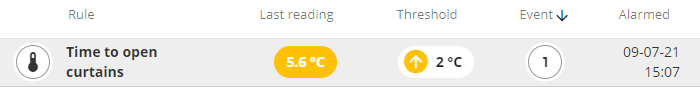What could be practical appliance of Expert virtual sensor in Aranet cloud?

•You can select an Expert template when creating a Virtual sensor in the Aranet cloud: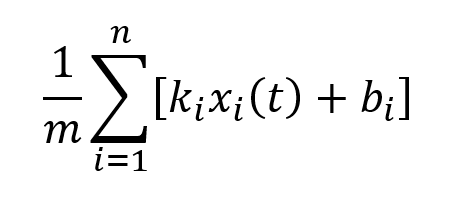This formula represents calculations to be made with measured data of up to 10 sensors. All input sensors must have the same metric. The result of calculations will have the same metric as input sensors.

Formula variables are:

• x(t) - input sensors measurement, unit is defined by sensor's configuration;
• k -  slope, a real number in the range of -1e+10 to 1e+10;
• b - offset, the same unit as input sensor;
• n - number of sensors, min = 1, max = 10;
• m - normalizing factor, a real number in the range of -1e+10 to 1e+10, must not be equal to 0.

Indeed, Offset, Average and Sum are special cases of  Expert formula.

Simple implementation of this formula can give you a more precise value of, f.e. average temperature in the room. Let's take for example a room where 3 pcs. of individually calibrated temperature sensors are placed: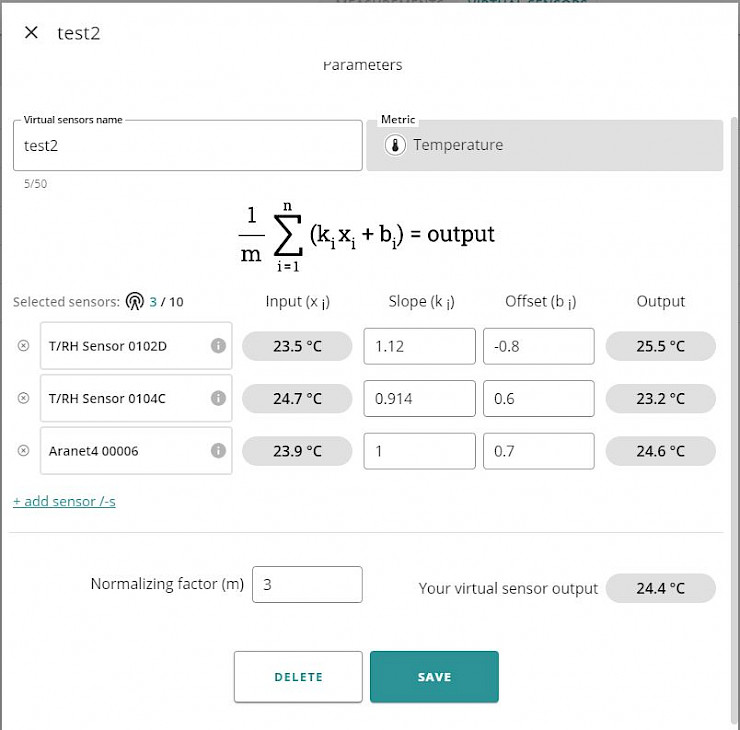Sensors offsets values were obtained experimentally by comparing measured data with laboratory-grade thermometer data.

Sensor #1 has offset b2 = -0.8° and is placed near Air Conditioner, so its measurements are affected by cold airflow and should be corrected by slope, let's say k2 = 1.12.

Sensor #2 has offset b1 = 0.6° and is placed on the sunny side of the room, so in the daytime, its measured temperature is affected by sun radiation and should be corrected by slope, let's say k1 = 0.914.

Sensor #3 has offset b3 = 0.7° and is placed in the centre of the room and its measurements are not affected by external influences, so the slope is k3 = 1.

In this case consider m = n = 3, last measurements readings are visible in the input column: x1 = 23.5, x2 = 24.7, x3 = 23.9. And calculated virtual sensor out is 24.4°. This value can be considered as rooms average temperature.

•As outdoors is very hot and I do not have aircon, I have created a virtual sensor that measures delta between indoor and outdoor temperature and if it goes below or above the thresholds I get a notification to open or close the window to preserve room temperature as low as possible.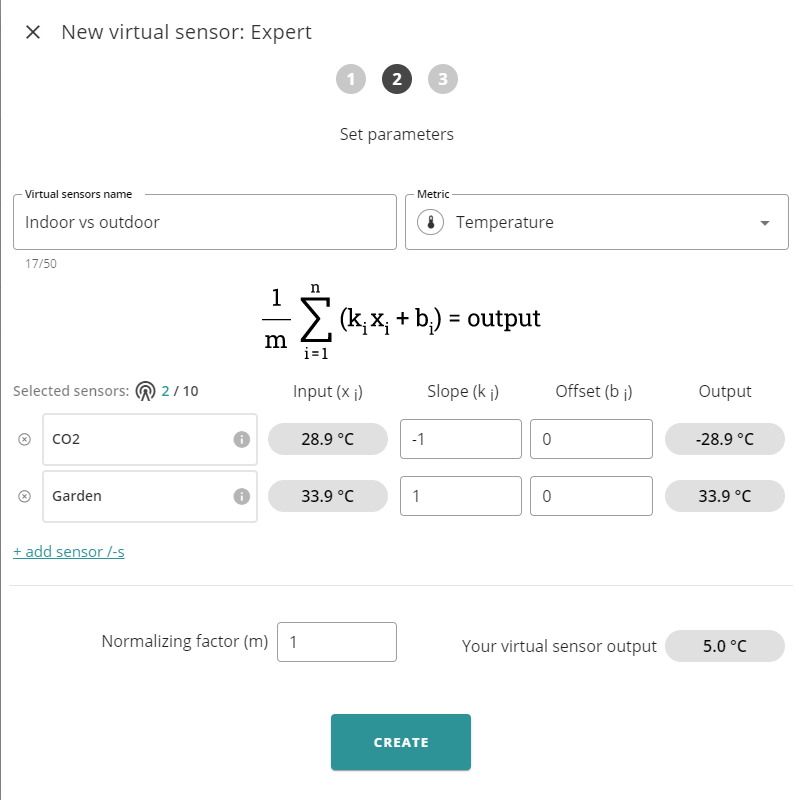Virtual sensor to create delta - now outdoors is close to 34 degrees Celsius hot and the room is 28.9, so I use -1 as a multiplier to calculate the delta.
The second step is Alarm based on the virtual sensor: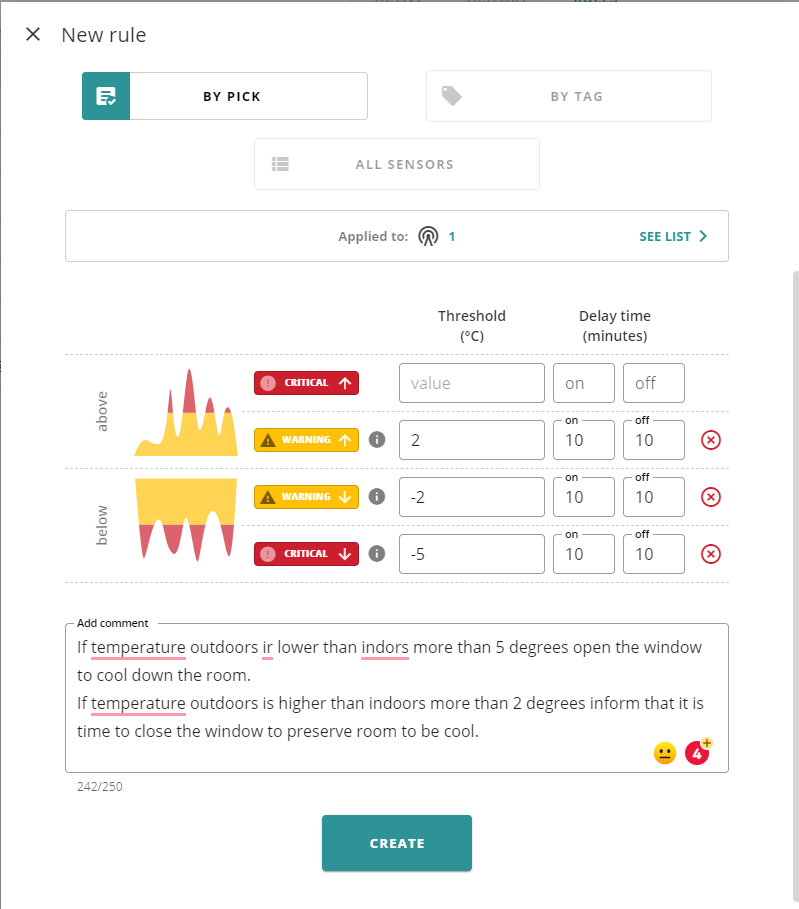Result - if the outside is hotter than indoors at least 2 degrees I am notified to close the window and preserve the room in mild condition.  when during evening temperature drops below room temperature I get notifications to open the window and cool the room a natural way.

And this is how do I see it in notifications - Alarm is active as delta is above 2 degrees